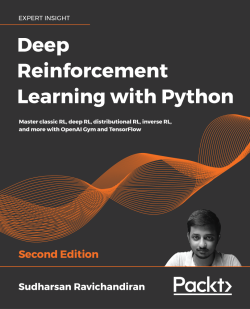### Deep Reinforcement Learning with Python - Second Edition

By Sudharsan Ravichandiran
• 7-day trial Subscribe Access now
• \$39.99 Print + eBook
• \$27.99 eBook

• Constantly updated with 100+ new titles each month
• Breadth and depth in over 1,000+ technologies

With significant enhancements in the quality and quantity of algorithms in recent years, this second edition of Hands-On Reinforcement Learning with Python has been revamped into an example-rich guide to learning state-of-the-art reinforcement learning (RL) and deep RL algorithms with TensorFlow 2 and the OpenAI Gym toolkit.

In addition to exploring RL basics and foundational concepts such as Bellman equation, Markov decision processes, and dynamic programming algorithms, this second edition dives deep into the full spectrum of value-based, policy-based, and actor-critic RL methods. It explores state-of-the-art algorithms such as DQN, TRPO, PPO and ACKTR, DDPG, TD3, and SAC in depth, demystifying the underlying math and demonstrating implementations through simple code examples.

The book has several new chapters dedicated to new RL techniques, including distributional RL, imitation learning, inverse RL, and meta RL. You will learn to leverage stable baselines, an improvement of OpenAI’s baseline library, to effortlessly implement popular RL algorithms. The book concludes with an overview of promising approaches such as meta-learning and imagination augmented agents in research.

By the end, you will become skilled in effectively employing RL and deep RL in your real-world projects.

Publication date:
September 2020
Publisher
Packt
Pages
760
ISBN
9781839210686

# Fundamentals of Reinforcement Learning

Reinforcement Learning (RL) is one of the areas of Machine Learning (ML). Unlike other ML paradigms, such as supervised and unsupervised learning, RL works in a trial and error fashion by interacting with its environment.

RL is one of the most active areas of research in artificial intelligence, and it is believed that RL will take us a step closer towards achieving artificial general intelligence. RL has evolved rapidly in the past few years with a wide variety of applications ranging from building a recommendation system to self-driving cars. The major reason for this evolution is the advent of deep reinforcement learning, which is a combination of deep learning and RL. With the emergence of new RL algorithms and libraries, RL is clearly one of the most promising areas of ML.

In this chapter, we will build a strong foundation in RL by exploring several important and fundamental concepts involved in RL.

In this chapter, we will cover the following topics:

• Key elements of RL
• The basic idea of RL
• The RL algorithm
• How RL differs from other ML paradigms
• The Markov Decision Processes
• Fundamental concepts of RL
• Applications of RL
• RL glossary

We will begin the chapter by defining Key elements of RL. This will help explain The basic idea of RL.

# Key elements of RL

Let's begin by understanding some key elements of RL.

## Agent

An agent is a software program that learns to make intelligent decisions. We can say that an agent is a learner in the RL setting. For instance, a chess player can be considered an agent since the player learns to make the best moves (decisions) to win the game. Similarly, Mario in a Super Mario Bros video game can be considered an agent since Mario explores the game and learns to make the best moves in the game.

## Environment

The environment is the world of the agent. The agent stays within the environment. For instance, coming back to our chess game, a chessboard is called the environment since the chess player (agent) learns to play the game of chess within the chessboard (environment). Similarly, in Super Mario Bros, the world of Mario is called the environment.

## State and action

A state is a position or a moment in the environment that the agent can be in. We learned that the agent stays within the environment, and there can be many positions in the environment that the agent can stay in, and those positions are called states. For instance, in our chess game example, each position on the chessboard is called the state. The state is usually denoted by s.

The agent interacts with the environment and moves from one state to another by performing an action. In the chess game environment, the action is the move performed by the player (agent). The action is usually denoted by a.

## Reward

We learned that the agent interacts with an environment by performing an action and moves from one state to another. Based on the action, the agent receives a reward. A reward is nothing but a numerical value, say, +1 for a good action and -1 for a bad action. How do we decide if an action is good or bad?

In our chess game example, if the agent makes a move in which it takes one of the opponent's chess pieces, then it is considered a good action and the agent receives a positive reward. Similarly, if the agent makes a move that leads to the opponent taking the agent's chess piece, then it is considered a bad action and the agent receives a negative reward. The reward is denoted by r.

# The basic idea of RL

Let's begin with an analogy. Let's suppose we are teaching a dog (agent) to catch a ball. Instead of teaching the dog explicitly to catch a ball, we just throw a ball and every time the dog catches the ball, we give the dog a cookie (reward). If the dog fails to catch the ball, then we do not give it a cookie. So, the dog will figure out what action caused it to receive a cookie and repeat that action. Thus, the dog will understand that catching the ball caused it to receive a cookie and will attempt to repeat catching the ball. Thus, in this way, the dog will learn to catch a ball while aiming to maximize the cookies it can receive.

Similarly, in an RL setting, we will not teach the agent what to do or how to do it; instead, we will give a reward to the agent for every action it does. We will give a positive reward to the agent when it performs a good action and we will give a negative reward to the agent when it performs a bad action. The agent begins by performing a random action and if the action is good, we then give the agent a positive reward so that the agent understands it has performed a good action and it will repeat that action. If the action performed by the agent is bad, then we will give the agent a negative reward so that the agent will understand it has performed a bad action and it will not repeat that action.

Thus, RL can be viewed as a trial and error learning process where the agent tries out different actions and learns the good action, which gives a positive reward.

In the dog analogy, the dog represents the agent, and giving a cookie to the dog upon it catching the ball is a positive reward and not giving a cookie is a negative reward. So, the dog (agent) explores different actions, which are catching the ball and not catching the ball, and understands that catching the ball is a good action as it brings the dog a positive reward (getting a cookie).

Let's further explore the idea of RL with one more simple example. Let's suppose we want to teach a robot (agent) to walk without hitting a mountain, as Figure 1.1 shows:

Figure 1.1: Robot walking

We will not teach the robot explicitly to not go in the direction of the mountain. Instead, if the robot hits the mountain and gets stuck, we give the robot a negative reward, say -1. So, the robot will understand that hitting the mountain is the wrong action, and it will not repeat that action:

Figure 1.2: Robot hits mountain

Similarly, when the robot walks in the right direction without hitting the mountain, we give the robot a positive reward, say +1. So, the robot will understand that not hitting the mountain is a good action, and it will repeat that action:

Figure 1.3: Robot avoids mountain

Thus, in the RL setting, the agent explores different actions and learns the best action based on the reward it gets.

Now that we have a basic idea of how RL works, in the upcoming sections, we will go into more detail and also learn the important concepts involved in RL.

# The RL algorithm

The steps involved in a typical RL algorithm are as follows:

1. First, the agent interacts with the environment by performing an action.
2. By performing an action, the agent moves from one state to another.
3. Then the agent will receive a reward based on the action it performed.
4. Based on the reward, the agent will understand whether the action is good or bad.
5. If the action was good, that is, if the agent received a positive reward, then the agent will prefer performing that action, else the agent will try performing other actions in search of a positive reward.

RL is basically a trial and error learning process. Now, let's revisit our chess game example. The agent (software program) is the chess player. So, the agent interacts with the environment (chessboard) by performing an action (moves). If the agent gets a positive reward for an action, then it will prefer performing that action; else it will find a different action that gives a positive reward.

Ultimately, the goal of the agent is to maximize the reward it gets. If the agent receives a good reward, then it means it has performed a good action. If the agent performs a good action, then it implies that it can win the game. Thus, the agent learns to win the game by maximizing the reward.

## RL agent in the grid world

Let's strengthen our understanding of RL by looking at another simple example. Consider the following grid world environment:

Figure 1.4: Grid world environment

The positions A to I in the environment are called the states of the environment. The goal of the agent is to reach state I by starting from state A without visiting the shaded states (B, C, G, and H). Thus, in order to achieve the goal, whenever our agent visits a shaded state, we will give a negative reward (say -1) and when it visits an unshaded state, we will give a positive reward (say +1). The actions in the environment are moving up, down, right and left. The agent can perform any of these four actions to reach state I from state A.

The first time the agent interacts with the environment (the first iteration), the agent is unlikely to perform the correct action in each state, and thus it receives a negative reward. That is, in the first iteration, the agent performs a random action in each state, and this may lead the agent to receive a negative reward. But over a series of iterations, the agent learns to perform the correct action in each state through the reward it obtains, helping it achieve the goal. Let us explore this in detail.

Iteration 1

As we learned, in the first iteration, the agent performs a random action in each state. For instance, look at the following figure. In the first iteration, the agent moves right from state A and reaches the new state B. But since B is the shaded state, the agent will receive a negative reward and so the agent will understand that moving right is not a good action in state A. When it visits state A next time, it will try out a different action instead of moving right:

Figure 1.5: Actions taken by the agent in iteration 1

As Figure 1.5 shows, from state B, the agent moves down and reaches the new state E. Since E is an unshaded state, the agent will receive a positive reward, so the agent will understand that moving down from state B is a good action.

From state E, the agent moves right and reaches state F. Since F is an unshaded state, the agent receives a positive reward, and it will understand that moving right from state E is a good action. From state F, the agent moves down and reaches the goal state I and receives a positive reward, so the agent will understand that moving down from state F is a good action.

Iteration 2

In the second iteration, from state A, instead of moving right, the agent tries out a different action as the agent learned in the previous iteration that moving right is not a good action in state A.

Thus, as Figure 1.6 shows, in this iteration the agent moves down from state A and reaches state D. Since D is an unshaded state, the agent receives a positive reward and now the agent will understand that moving down is a good action in state A:

Figure 1.6: Actions taken by the agent in iteration 2

As shown in the preceding figure, from state D, the agent moves down and reaches state G. But since G is a shaded state, the agent will receive a negative reward and so the agent will understand that moving down is not a good action in state D, and when it visits state D next time, it will try out a different action instead of moving down.

From G, the agent moves right and reaches state H. Since H is a shaded state, it will receive a negative reward and understand that moving right is not a good action in state G.

From H it moves right and reaches the goal state I and receives a positive reward, so the agent will understand that moving right from state H is a good action.

Iteration 3

In the third iteration, the agent moves down from state A since, in the second iteration, our agent learned that moving down is a good action in state A. So, the agent moves down from state A and reaches the next state, D, as Figure 1.7 shows:

Figure 1.7: Actions taken by the agent in iteration 3

Now, from state D, the agent tries a different action instead of moving down since in the second iteration our agent learned that moving down is not a good action in state D. So, in this iteration, the agent moves right from state D and reaches state E.

From state E, the agent moves right as the agent already learned in the first iteration that moving right from state E is a good action and reaches state F.

Now, from state F, the agent moves down since the agent learned in the first iteration that moving down is a good action in state F, and reaches the goal state I.

Figure 1.8 shows the result of the third iteration:

Figure 1.8: The agent reaches the goal state without visiting the shaded states

As we can see, our agent has successfully learned to reach the goal state I from state A without visiting the shaded states based on the rewards.

In this way, the agent will try out different actions in each state and understand whether an action is good or bad based on the reward it obtains. The goal of the agent is to maximize rewards. So, the agent will always try to perform good actions that give a positive reward, and when the agent performs good actions in each state, then it ultimately leads the agent to achieve the goal.

Note that these iterations are called episodes in RL terminology. We will learn more about episodes later in the chapter.

# How RL differs from other ML paradigms

We can categorize ML into three types:

• Supervised learning
• Unsupervised learning
• RL

In supervised learning, the machine learns from training data. The training data consists of a labeled pair of inputs and outputs. So, we train the model (agent) using the training data in such a way that the model can generalize its learning to new unseen data. It is called supervised learning because the training data acts as a supervisor, since it has a labeled pair of inputs and outputs, and it guides the model in learning the given task.

Now, let's understand the difference between supervised and reinforcement learning with an example. Consider the dog analogy we discussed earlier in the chapter. In supervised learning, to teach the dog to catch a ball, we will teach it explicitly by specifying turn left, go right, move forward seven steps, catch the ball, and so on in the form of training data. But in RL, we just throw a ball, and every time the dog catches the ball, we give it a cookie (reward). So, the dog will learn to catch the ball while trying to maximize the cookies (reward) it can get.

Let's consider one more example. Say we want to train the model to play chess using supervised learning. In this case, we will have training data that includes all the moves a player can make in each state, along with labels indicating whether it is a good move or not. Then, we train the model to learn from this training data, whereas in the case of RL, our agent will not be given any sort of training data; instead, we just give a reward to the agent for each action it performs. Then, the agent will learn by interacting with the environment and, based on the reward it gets, it will choose its actions.

Similar to supervised learning, in unsupervised learning, we train the model (agent) based on the training data. But in the case of unsupervised learning, the training data does not contain any labels; that is, it consists of only inputs and not outputs. The goal of unsupervised learning is to determine hidden patterns in the input. There is a common misconception that RL is a kind of unsupervised learning, but it is not. In unsupervised learning, the model learns the hidden structure, whereas, in RL, the model learns by maximizing the reward.

For instance, consider a movie recommendation system. Say we want to recommend a new movie to the user. With unsupervised learning, the model (agent) will find movies similar to the movies the user (or users with a profile similar to the user) has viewed before and recommend new movies to the user.

With RL, the agent constantly receives feedback from the user. This feedback represents rewards (a reward could be ratings the user has given for a movie they have watched, time spent watching a movie, time spent watching trailers, and so on). Based on the rewards, an RL agent will understand the movie preference of the user and then suggest new movies accordingly.

Since the RL agent is learning with the aid of rewards, it can understand if the user's movie preference changes and suggest new movies according to the user's changed movie preference dynamically.

Thus, we can say that in both supervised and unsupervised learning the model (agent) learns based on the given training dataset, whereas in RL the agent learns by directly interacting with the environment. Thus, RL is essentially an interaction between the agent and its environment.

Before moving on to the fundamental concepts of RL, we will introduce a popular process to aid decision-making in an RL environment.

# Markov Decision Processes

The Markov Decision Process (MDP) provides a mathematical framework for solving the RL problem. Almost all RL problems can be modeled as an MDP. MDPs are widely used for solving various optimization problems. In this section, we will understand what an MDP is and how it is used in RL.

To understand an MDP, first, we need to learn about the Markov property and Markov chain.

## The Markov property and Markov chain

The Markov property states that the future depends only on the present and not on the past. The Markov chain, also known as the Markov process, consists of a sequence of states that strictly obey the Markov property; that is, the Markov chain is the probabilistic model that solely depends on the current state to predict the next state and not the previous states, that is, the future is conditionally independent of the past.

For example, if we want to predict the weather and we know that the current state is cloudy, we can predict that the next state could be rainy. We concluded that the next state is likely to be rainy only by considering the current state (cloudy) and not the previous states, which might have been sunny, windy, and so on.

However, the Markov property does not hold for all processes. For instance, throwing a dice (the next state) has no dependency on the previous number that showed up on the dice (the current state).

Moving from one state to another is called a transition, and its probability is called a transition probability. We denote the transition probability by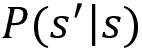. It indicates the probability of moving from the state s to the next state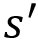. Say we have three states (cloudy, rainy, and windy) in our Markov chain. Then we can represent the probability of transitioning from one state to another using a table called a Markov table, as shown in Table 1.1:

Table 1.1: An example of a Markov table

From Table 1.1, we can observe that:

• From the state cloudy, we transition to the state rainy with 70% probability and to the state windy with 30% probability.
• From the state rainy, we transition to the same state rainy with 80% probability and to the state cloudy with 20% probability.
• From the state windy, we transition to the state rainy with 100% probability.

We can also represent this transition information of the Markov chain in the form of a state diagram, as shown in Figure 1.9:

Figure 1.9: A state diagram of a Markov chain

We can also formulate the transition probabilities into a matrix called the transition matrix, as shown in Figure 1.10:

Figure 1.10: A transition matrix

Thus, to conclude, we can say that the Markov chain or Markov process consists of a set of states along with their transition probabilities.

## The Markov Reward Process

The Markov Reward Process (MRP) is an extension of the Markov chain with the reward function. That is, we learned that the Markov chain consists of states and a transition probability. The MRP consists of states, a transition probability, and also a reward function.

A reward function tells us the reward we obtain in each state. For instance, based on our previous weather example, the reward function tells us the reward we obtain in the state cloudy, the reward we obtain in the state windy, and so on. The reward function is usually denoted by R(s).

Thus, the MRP consists of states s, a transition probability, and a reward function R(s).

## The Markov Decision Process

The Markov Decision Process (MDP) is an extension of the MRP with actions. That is, we learned that the MRP consists of states, a transition probability, and a reward function. The MDP consists of states, a transition probability, a reward function, and also actions. We learned that the Markov property states that the next state is dependent only on the current state and is not based on the previous state. Is the Markov property applicable to the RL setting? Yes! In the RL environment, the agent makes decisions only based on the current state and not based on the past states. So, we can model an RL environment as an MDP.

Let's understand this with an example. Given any environment, we can formulate the environment using an MDP. For instance, let's consider the same grid world environment we learned earlier. Figure 1.11 shows the grid world environment, and the goal of the agent is to reach state I from state A without visiting the shaded states:

Figure 1.11: Grid world environment

An agent makes a decision (action) in the environment only based on the current state the agent is in and not based on the past state. So, we can formulate our environment as an MDP. We learned that the MDP consists of states, actions, transition probabilities, and a reward function. Now, let's learn how this relates to our RL environment:

States – A set of states present in the environment. Thus, in the grid world environment, we have states A to I.

Actions – A set of actions that our agent can perform in each state. An agent performs an action and moves from one state to another. Thus, in the grid world environment, the set of actions is up, down, left, and right.

Transition probability – The transition probability is denoted by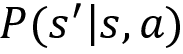. It implies the probability of moving from a state s to the next statewhile performing an action a. If you observe, in the MRP, the transition probability is just, that is, the probability of going from state s to state, and it doesn't include actions. But in the MDP, we include the actions, and thus the transition probability is denoted by.

For example, in our grid world environment, say the transition probability of moving from state A to state B while performing an action right is 100%. This can be expressed as P(B|A, right) = 1.0. We can also view this in the state diagram, as shown in Figure 1.12:

Figure 1.12: Transition probability of moving right from A to B

Suppose our agent is in state C and the transition probability of moving from state C to state F while performing the action down is 90%, then it can be expressed as P(F|C, down) = 0.9. We can also view this in the state diagram, as shown in Figure 1.13:

Figure 1.13: Transition probability of moving down from C to F

Reward function - The reward function is denoted by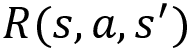. It represents the reward our agent obtains while transitioning from state s to statewhile performing an action a.

Say the reward we obtain while transitioning from state A to state B while performing the action right is -1, then it can be expressed as R(A, right, B) = -1. We can also view this in the state diagram, as shown in Figure 1.14:

Figure 1.14: Reward of moving right from A to B

Suppose our agent is in state C and say the reward we obtain while transitioning from state C to state F while performing the action down is +1, then it can be expressed as R(C, down, F) = +1. We can also view this in the state diagram, as shown in Figure 1.15:

Figure 1.15: Reward of moving down from C to F

Thus, an RL environment can be represented as an MDP with states, actions, transition probability, and the reward function. But wait! What is the use of representing the RL environment using the MDP? We can solve the RL problem easily once we model our environment as the MDP. For instance, once we model our grid world environment using the MDP, then we can easily find how to reach the goal state I from state A without visiting the shaded states. We will learn more about this in the upcoming chapters. Next, we will go through more essential concepts of RL.

# Fundamental concepts of RL

In this section, we will learn about several important fundamental RL concepts.

## Math essentials

Before going ahead, let's quickly recap expectation from our high school days, as we will be dealing with expectation throughout the book.

### Expectation

Let's say we have a variable X and it has the values 1, 2, 3, 4, 5, 6. To compute the average value of X, we can just sum all the values of X divided by the number of values of X. Thus, the average of X is (1+2+3+4+5+6)/6 = 3.5.

Now, let's suppose X is a random variable. The random variable takes values based on a random experiment, such as throwing dice, tossing a coin, and so on. The random variable takes different values with some probabilities. Let's suppose we are throwing a fair dice, then the possible outcomes (X) are 1, 2, 3, 4, 5, and 6 and the probability of occurrence of each of these outcomes is 1/6, as shown in Table 1.2:

Table 1.2: Probabilities of throwing a dice

How can we compute the average value of the random variable X? Since each value has a probability of an occurrence, we can't just take the average. So, instead, we compute the weighted average, that is, the sum of values of X multiplied by their respective probabilities, and this is called expectation. The expectation of a random variable X can be defined as:

Thus, the expectation of the random variable X is E(X) = 1(1/6) + 2(1/6) + 3(1/6) + 4(1/6) + 5 (1/6) + 6(1/6) = 3.5.

The expectation is also known as the expected value. Thus, the expected value of the random variable X is 3.5. Thus, when we say expectation or the expected value of a random variable, it basically means the weighted average.

Now, we will look into the expectation of a function of a random variable. Let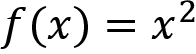, then we can write:

Table 1.3: Probabilities of throwing a dice

The expectation of a function of a random variable can be computed as:

Thus, the expected value of f(X) is given as E(f(X)) = 1(1/6) + 4(1/6) + 9(1/6) + 16(1/6) + 25(1/6) + 36(1/6) = 15.1.

## Action space

Consider the grid world environment shown in Figure 1.16:

Figure 1.16: Grid world environment

In the preceding grid world environment, the goal of the agent is to reach state I starting from state A without visiting the shaded states. In each of the states, the agent can perform any of the four actions—up, down, left, and right—to achieve the goal. The set of all possible actions in the environment is called the action space. Thus, for this grid world environment, the action space will be [up, down, left, right].

We can categorize action spaces into two types:

• Discrete action space
• Continuous action space

Discrete action space: When our action space consists of actions that are discrete, then it is called a discrete action space. For instance, in the grid world environment, our action space consists of four discrete actions, which are up, down, left, right, and so it is called a discrete action space.

Continuous action space: When our action space consists of actions that are continuous, then it is called a continuous action space. For instance, let's suppose we are training an agent to drive a car, then our action space will consist of several actions that have continuous values, such as the speed at which we need to drive the car, the number of degrees we need to rotate the wheel, and so on. In cases where our action space consists of actions that are continuous, it is called a continuous action space.

## Policy

A policy defines the agent's behavior in an environment. The policy tells the agent what action to perform in each state. For instance, in the grid world environment, we have states A to I and four possible actions. The policy may tell the agent to move down in state A, move right in state D, and so on.

To interact with the environment for the first time, we initialize a random policy, that is, the random policy tells the agent to perform a random action in each state. Thus, in an initial iteration, the agent performs a random action in each state and tries to learn whether the action is good or bad based on the reward it obtains. Over a series of iterations, an agent will learn to perform good actions in each state, which gives a positive reward. Thus, we can say that over a series of iterations, the agent will learn a good policy that gives a positive reward.

This good policy is called the optimal policy. The optimal policy is the policy that gets the agent a good reward and helps the agent to achieve the goal. For instance, in our grid world environment, the optimal policy tells the agent to perform an action in each state such that the agent can reach state I from state A without visiting the shaded states.

The optimal policy is shown in Figure 1.17. As we can observe, the agent selects the action in each state based on the optimal policy and reaches the terminal state I from the starting state A without visiting the shaded states:

Figure 1.17: The optimal policy in the grid world environment

Thus, the optimal policy tells the agent to perform the correct action in each state so that the agent can receive a good reward.

A policy can be classified as the following:

• A deterministic policy
• A stochastic policy

### Deterministic policy

The policy that we just covered is called a deterministic policy. A deterministic policy tells the agent to perform one particular action in a state. Thus, the deterministic policy maps the state to one particular action and is often denoted by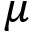. Given a state s at a time t, a deterministic policy tells the agent to perform one particular action a. It can be expressed as:

For instance, consider our grid world example. Given state A, the deterministic policytells the agent to perform the action down. This can be expressed as:

Thus, according to the deterministic policy, whenever the agent visits state A, it performs the action down.

### Stochastic policy

Unlike a deterministic policy, a stochastic policy does not map a state directly to one particular action; instead, it maps the state to a probability distribution over an action space.

That is, we learned that given a state, the deterministic policy will tell the agent to perform one particular action in the given state, so whenever the agent visits the state it always performs the same particular action. But with a stochastic policy, given a state, the stochastic policy will return a probability distribution over an action space. So instead of performing the same action every time the agent visits the state, the agent performs different actions each time based on a probability distribution returned by the stochastic policy.

Let's understand this with an example; we know that our grid world environment's action space consists of four actions, which are [up, down, left, right]. Given a state A, the stochastic policy returns the probability distribution over the action space as [0.10,0.70,0.10,0.10]. Now, whenever the agent visits state A, instead of selecting the same particular action every time, the agent selects up 10% of the time, down 70% of the time, left 10% of the time, and right 10% of the time.

The difference between the deterministic policy and stochastic policy is shown in Figure 1.18. As we can observe, the deterministic policy maps the state to one particular action, whereas the stochastic policy maps the state to the probability distribution over an action space:

Figure 1.18: The difference between deterministic and stochastic policies

Thus, the stochastic policy maps the state to a probability distribution over the action space and is often denoted by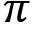. Say we have a state s and action a at a time t, then we can express the stochastic policy as:

Or it can also be expressed as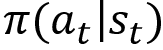.

We can categorize the stochastic policy into two types:

• Categorical policy
• Gaussian policy

#### Categorical policy

A stochastic policy is called a categorical policy when the action space is discrete. That is, the stochastic policy uses a categorical probability distribution over the action space to select actions when the action space is discrete. For instance, in the grid world environment from the previous example, we select actions based on a categorical probability distribution (discrete distribution) as the action space of the environment is discrete. As Figure 1.19 shows, given state A, we select an action based on the categorical probability distribution over the action space:

Figure 1.19: Probability of next move from state A for a discrete action space

#### Gaussian policy

A stochastic policy is called a Gaussian policy when our action space is continuous. That is, the stochastic policy uses a Gaussian probability distribution over the action space to select actions when the action space is continuous. Let's understand this with a simple example. Suppose we are training an agent to drive a car and say we have one continuous action in our action space. Let the action be the speed of the car, and the value of the speed of the car ranges from 0 to 150 kmph. Then, the stochastic policy uses the Gaussian distribution over the action space to select an action, as Figure 1.20 shows:

Figure 1.20: Gaussian distribution

## Episode

The agent interacts with the environment by performing some actions, starting from the initial state and reaches the final state. This agent-environment interaction starting from the initial state until the final state is called an episode. For instance, in a car racing video game, the agent plays the game by starting from the initial state (the starting point of the race) and reaches the final state (the endpoint of the race). This is considered an episode. An episode is also often called a trajectory (the path taken by the agent) and it is denoted by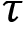.

An agent can play the game for any number of episodes, and each episode is independent of the others. What is the use of playing the game for multiple episodes? In order to learn the optimal policy, that is, the policy that tells the agent to perform the correct action in each state, the agent plays the game for many episodes.

For example, let's say we are playing a car racing game; the first time, we may not win the game, so we play the game several times to understand more about the game and discover some good strategies for winning the game. Similarly, in the first episode, the agent may not win the game and it plays the game for several episodes to understand more about the game environment and good strategies to win the game.

Say we begin the game from an initial state at a time step t = 0 and reach the final state at a time step T, then the episode information consists of the agent-environment interaction, such as state, action, and reward, starting from the initial state until the final state, that is, (s0, a0, r0, s1, a1, r1,…,sT).

Figure 1.21 shows an example of an episode/trajectory:

Figure 1.21: An example of an episode

Let's strengthen our understanding of the episode and the optimal policy with the grid world environment. We learned that in the grid world environment, the goal of our agent is to reach the final state I starting from the initial state A without visiting the shaded states. An agent receives a +1 reward when it visits the unshaded states and a -1 reward when it visits the shaded states.

When we say generate an episode, it means going from the initial state to the final state. The agent generates the first episode using a random policy and explores the environment and over several episodes, it will learn the optimal policy.

Episode 1

As the Figure 1.22 shows, in the first episode, the agent uses a random policy and selects a random action in each state from the initial state until the final state and observes the reward:

Figure 1.22: Episode 1

Episode 2

In the second episode, the agent tries a different policy to avoid the negative rewards it received in the previous episode. For instance, as we can observe in the previous episode, the agent selected the action right in state A and received a negative reward, so in this episode, instead of selecting the action right in state A, it tries a different action, say down, as shown in Figure 1.23:

Figure 1.23: Episode 2

Episode n

Thus, over a series of episodes, the agent learns the optimal policy, that is, the policy that takes the agent to the final state I from state A without visiting the shaded states, as Figure 1.24 shows:

Figure 1.24: Episode n

An RL task can be categorized as:

Episodic task: As the name suggests, an episodic task is one that has a terminal/final state. That is, episodic tasks are tasks made up of episodes and thus they have a terminal state. For example, in a car racing game, we start from the starting point (initial state) and reach the destination (terminal state).

Continuous task: Unlike episodic tasks, continuous tasks do not contain any episodes and so they don't have any terminal state. For example, a personal assistance robot does not have a terminal state.

## Horizon

Horizon is the time step until which the agent interacts with the environment. We can classify the horizon into two categories:

• Finite horizon
• Infinite horizon

Finite horizon: If the agent-environment interaction stops at a particular time step, then the horizon is called a finite horizon. For instance, in episodic tasks, an agent interacts with the environment by starting from the initial state at time step t = 0 and reaches the final state at time step T. Since the agent-environment interaction stops at time step T, it is considered a finite horizon.

Infinite horizon: If the agent-environment interaction never stops, then it is called an infinite horizon. For instance, we learned that a continuous task has no terminal states. This means the agent-environment interaction will never stop in a continuous task and so it is considered an infinite horizon.

## Return and discount factor

A return can be defined as the sum of the rewards obtained by the agent in an episode. The return is often denoted by R or G. Say the agent starts from the initial state at time step t = 0 and reaches the final state at time step T, then the return obtained by the agent is given as:

Let's understand this with an example; consider the trajectory (episode):

Figure 1.25: Trajectory/episodeThe return of the trajectory is the sum of the rewards, that is,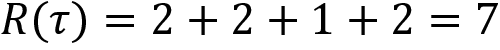.

Thus, we can say that the goal of our agent is to maximize the return, that is, maximize the sum of rewards (cumulative rewards) obtained over the episode. How can we maximize the return? We can maximize the return if we perform the correct action in each state. Okay, how can we perform the correct action in each state? We can perform the correct action in each state by using the optimal policy. Thus, we can maximize the return using the optimal policy. Thus, the optimal policy is the policy that gets our agent the maximum return (sum of rewards) by performing the correct action in each state.

Okay, how can we define the return for continuous tasks? We learned that in continuous tasks there are no terminal states, so we can define the return as a sum of rewards up to infinity:

But how can we maximize the return that just sums to infinity? We introduce a new term called discount factor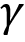and rewrite our return as:

Okay, but how is this discount factor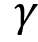helping us? It helps us in preventing the return from reaching infinity by deciding how much importance we give to future rewards and immediate rewards. The value of the discount factor ranges from 0 to 1. When we set the discount factor to a small value (close to 0), it implies that we give more importance to immediate rewards than to future rewards. When we set the discount factor to a high value (close to 1), it implies that we give more importance to future rewards than to immediate rewards. Let's understand this with an example with different discount factor values.

### Small discount factor

Let's set the discount factor to a small value, say 0.2, that is, let's set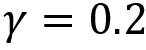, then we can write:

From this equation, we can observe that the reward at each time step is weighted by a discount factor. As the time steps increase, the discount factor (weight) decreases and thus the importance of rewards at future time steps also decreases. That is, from the equation, we can observe that:

• At time step 0, the reward r0 is weighted by a discount factor of 1.
• At time step 1, the reward r1 is weighted by a heavily decreased discount factor of 0.2.
• At time step 2, the reward r2 is weighted by a heavily decreased discount factor of 0.04.

As we can observe, the discount factor is heavily decreased for the subsequent time steps and more importance is given to the immediate reward r0 than the rewards obtained at the future time steps. Thus, when we set the discount factor to a small value, we give more importance to the immediate reward than future rewards.

### Large discount factor

Let's set the discount factor to a high value, say 0.9, that is, let's set,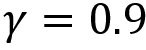, then we can write:

From this equation, we can infer that as the time step increases the discount factor (weight) decreases; however, it is not decreasing heavily (unlike the previous case) since here we started off with. So, in this case, we can say that we give more importance to future rewards. That is, from the equation, we can observe that:

• At time step 0, the reward r0 is weighted by a discount factor of 1.
• At time step 1, the reward r1 is weighted by a slightly decreased discount factor of 0.9.
• At time step 2, the reward r2 is weighted by a slightly decreased discount factor of 0.81.

As we can observe, the discount factor is decreased for subsequent time steps but unlike the previous case, the discount factor is not decreased heavily. Thus, when we set the discount factor to a high value, we give more importance to future rewards than the immediate reward.

### What happens when we set the discount factor to 0?

When we set the discount factor to 0, that is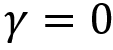, it implies that we consider only the immediate reward r0 and not the reward obtained from the future time steps. Thus, when we set the discount factor to 0, then the agent will never learn as it will consider only the immediate reward r0, as shown here:

As we can observe, when we set, our return will be just the immediate reward r0.

### What happens when we set the discount factor to 1?

When we set the discount factor to 1, that is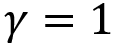, it implies that we consider all the future rewards. Thus, when we set the discount factor to 1, then the agent will learn forever, looking for all the future rewards, which may lead to infinity, as shown here:

As we can observe, when we set, then our return will be the sum of rewards up to infinity.

Thus, we have learned that when we set the discount factor to 0, the agent will never learn, considering only the immediate reward, and when we set the discount factor to 1 the agent will learn forever, looking for the future rewards that lead to infinity. So, the optimal value of the discount factor lies between 0.2 and 0.8.

But the question is, why should we care about immediate and future rewards? We give importance to immediate and future rewards depending on the tasks. In some tasks, future rewards are more desirable than immediate rewards, and vice versa. In a chess game, the goal is to defeat the opponent's king. If we give more importance to the immediate reward, which is acquired by actions such as our pawn defeating any opposing chessman, then the agent will learn to perform this sub-goal instead of learning the actual goal. So, in this case, we give greater importance to future rewards than the immediate reward, whereas in some cases, we prefer immediate rewards over future rewards. Would you prefer chocolates if I gave them to you today or 13 days later?

In the following two sections, we'll analyze the two fundamental functions of RL.

## The value function

The value function, also called the state value function, denotes the value of the state. The value of a state is the return an agent would obtain starting from that state following policy. The value of a state or value function is usually denoted by V(s) and it can be expressed as:

where s0 = s implies that the starting state is s. The value of a state is called the state value.

Let's understand the value function with an example. Let's suppose we generate the trajectoryfollowing some policyin our grid world environment, as shown in Figure 1.26:

Figure 1.26: A value function example

Now, how do we compute the value of all the states in our trajectory? We learned that the value of a state is the return (sum of rewards) an agent would obtain starting from that state following policy. The preceding trajectory is generated using policy, thus we can say that the value of a state is the return (sum of rewards) of the trajectory starting from that state:

• The value of state A is the return of the trajectory starting from state A. Thus, V(A) = 1+1+ -1+1 = 2.
• The value of state D is the return of the trajectory starting from state D. Thus, V(D) = 1-1+1= 1.
• The value of state E is the return of the trajectory starting from state E. Thus, V(E) = -1+1 = 0.
• The value of state H is the return of the trajectory starting from state H. Thus, V(H) = 1.

What about the value of the final state I? We learned the value of a state is the return (sum of rewards) starting from that state. We know that we obtain a reward when we transition from one state to another. Since I is the final state, we don't make any transition from the final state, so there is no reward and thus no value for the final state I.

In a nutshell, the value of a state is the return of the trajectory starting from that state.

Wait! There is a small change here: instead of taking the return directly as a value of a state, we will use the expected return. Thus, the value function or the value of state s can be defined as the expected return that the agent would obtain starting from state s following policy. It can be expressed as:

Now, the question is why expected return? Why we can't we just compute the value of a state as a return directly? Because our return is the random variable and it takes different values with some probability.

Let's understand this with a simple example. Suppose we have a stochastic policy. We learned that unlike the deterministic policy, which maps the state to the action directly, the stochastic policy maps the state to the probability distribution over the action space. Thus, the stochastic policy selects actions based on a probability distribution.

Let's suppose we are in state A and the stochastic policy returns the probability distribution over the action space as [0.0,0.80,0.00,0.20]. It implies that with the stochastic policy, in state A, we perform the action down 80% of the time, that is,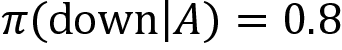, and the action right 20% of the time, that is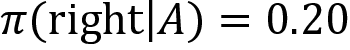.

Thus, in state A, our stochastic policyselects the action down 80% of the time and the action right 20% of the time, and say our stochastic policy selects the action right in states D and E and the action down in states B and F 100% of the time.

First, we generate an episode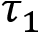using our stochastic policy, as shown in Figure 1.27:

Figure 1.27: EpisodeFor better understanding, let's focus only on the value of state A. The value of state A is the return (sum of rewards) of the trajectory starting from state A. Thus,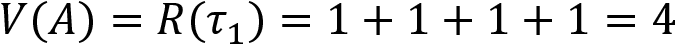.

Say we generate another episode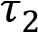using the same given stochastic policy, as shown in Figure 1.28:

Figure 1.28: EpisodeThe value of state A is the return (sum of rewards) of the trajectory from state A. Thus,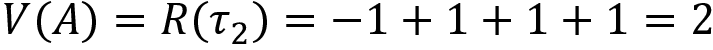.

As you may observe, although we use the same policy, the values of state A in trajectoriesandare different. This is because our policy is a stochastic policy and it performs the action down in state A 80% of the time and the action right in state A 20% of the time. So, when we generate a trajectory using policy, the trajectorywill occur 80% of the time and the trajectorywill occur 20% of the time. Thus, the return will be 4 for 80% of the time and 2 for 20% of the time.

Thus, instead of taking the value of the state as a return directly, we will take the expected return, since the return takes different values with some probability. The expected return is basically the weighted average, that is, the sum of the return multiplied by their probability. Thus, we can write:

The value of a state A can be obtained as:

Thus, the value of a state is the expected return of the trajectory starting from that state.

Note that the value function depends on the policy, that is, the value of the state varies based on the policy we choose. There can be many different value functions according to different policies. The optimal value function V*(s) yields the maximum value compared to all the other value functions. It can be expressed as:

For example, let's say we have two policies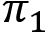and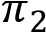. Let the value of state s using policybe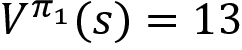and the value of state s using policybe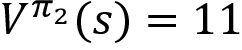. Then the optimal value of state s will be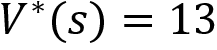as it is the maximum. The policy that gives the maximum state value is called the optimal policy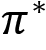. Thus, in this case,is the optimal policy as it gives the maximum state value.

We can view the value function in a table called a value table. Let's say we have two states s0 and s1, then the value function can be represented as:

Table 1.4: Value table

From the value table, we can tell that it is better to be in state s1 than state s0 as s1 has a higher value. Thus, we can say that state s1 is the optimal state.

## Q function

A Q function, also called the state-action value function, denotes the value of a state-action pair. The value of a state-action pair is the return the agent would obtain starting from state s and performing action a following policy. The value of a state-action pair or Q function is usually denoted by Q(s,a) and is known as the Q value or state-action value. It is expressed as:

Note that the only difference between the value function and Q function is that in the value function we compute the value of a state, whereas in the Q function we compute the value of a state-action pair. Let's understand the Q function with an example. Consider the trajectory in Figure 1.29 generated using policy:

Figure 1.29: A trajectory/episode example

We learned that the Q function computes the value of a state-action pair. Say we need to compute the Q value of state-action pair A-down. That is the Q value of moving down in state A. Then the Q value will be the return of our trajectory starting from state A and performing the action down:

Let's suppose we need to compute the Q value of the state-action pair D-right. That is the Q value of moving right in state D. The Q value will be the return of our trajectory starting from state D and performing the action right:

Similarly, we can compute the Q value for all the state-action pairs. Similar to what we learned about the value function, instead of taking the return directly as the Q value of a state-action pair, we use the expected return because the return is the random variable and it takes different values with some probability. So, we can redefine our Q function as:

It implies that the Q value is the expected return the agent would obtain starting from state s and performing action a following policy.

Similar to the value function, the Q function depends on the policy, that is, the Q value varies based on the policy we choose. There can be many different Q functions according to different policies. The optimal Q function is the one that has the maximum Q value over other Q functions, and it can be expressed as:

The optimal policyis the policy that gives the maximum Q value.

Like the value function, the Q function can be viewed in a table. It is called a Q table. Let's say we have two states s0 and s1, and two actions 0 and 1; then the Q function can be represented as follows:

Table 1.5: Q table

As we can observe, the Q table represents the Q values of all possible state-action pairs. We learned that the optimal policy is the policy that gets our agent the maximum return (sum of rewards). We can extract the optimal policy from the Q table by just selecting the action that has the maximum Q value in each state. Thus, our optimal policy will select action 1 in state s0 and action 0 in state s1 since they have a high Q value, as shown in Table 1.6:

Table 1.6: Optimal policy extracted from the Q table

Thus, we can extract the optimal policy by computing the Q function.

## Model-based and model-free learning

Now, let's look into two different types of learning called model-based and model-free learning.

Model-based learning: In model-based learning, an agent will have a complete description of the environment. We know that the transition probability tells us the probability of moving from state s to the next stateby performing action a. The reward function tells us the reward we would obtain while moving from state s to the next stateby performing action a. When the agent knows the model dynamics of its environment, that is, when the agent knows the transition probability of its environment, then the learning is called model-based learning. Thus, in model-based learning, the agent uses the model dynamics to find the optimal policy.

Model-free learning: Model-free learning is when the agent does not know the model dynamics of its environment. That is, in model-free learning, an agent tries to find the optimal policy without the model dynamics.

Next, we'll discover the different types of environment an agent works within.

## Different types of environments

At the beginning of the chapter, we learned that the environment is the world of the agent and the agent lives/stays within the environment. We can categorize the environment into different types.

### Deterministic and stochastic environments

Deterministic environment: In a deterministic environment, we are certain that when an agent performs action a in state s, then it always reaches state. For example, let's consider our grid world environment. Say the agent is in state A, and when it moves down from state A, it always reaches state D. Hence the environment is called a deterministic environment:

Figure 1.30: Deterministic environment

Stochastic environment: In a stochastic environment, we cannot say that by performing action a in state s the agent always reaches state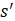because there will be some randomness associated with the stochastic environment. For example, let's suppose our grid world environment is a stochastic environment. Say our agent is in state A; now if it moves down from state A, then the agent doesn't always reach state D. Instead, it reaches state D 70% of the time and state B 30% of the time. That is, if the agent moves down in state A, then the agent reaches state D with 70% probability and state B with 30% probability, as Figure 1.31 shows:

Figure 1.31: Stochastic environment

### Discrete and continuous environments

Discrete environment: A discrete environment is one where the environment's action space is discrete. For instance, in the grid world environment, we have a discrete action space, which consists of the actions [up, down, left, right] and thus our grid world environment is discrete.

Continuous environment: A continuous environment is one where the environment's action space is continuous. For instance, suppose we are training an agent to drive a car, then our action space will be continuous, with several continuous actions such as changing the car's speed, the number of degrees the agent needs to rotate the wheel, and so on. In such a case, our environment's action space is continuous.

### Episodic and non-episodic environments

Episodic environment: In an episodic environment, an agent's current action will not affect future actions, and thus an episodic environment is also called a non-sequential environment.

Non-episodic environment: In a non-episodic environment, an agent's current action will affect future actions, and thus a non-episodic environment is also called a sequential environment. For example, a chessboard is a sequential environment since the agent's current action will affect future actions in a chess match.

### Single and multi-agent environments

• Single-agent environment: When our environment consists of only a single agent, then it is called a single-agent environment.
• Multi-agent environment: When our environment consists of multiple agents, then it is called a multi-agent environment.

We have covered a lot of concepts of RL. Now, we'll finish the chapter by looking at some exciting applications of RL.

# Applications of RL

RL has evolved rapidly over the past couple of years with a wide range of applications ranging from playing games to self-driving cars. One of the major reasons for this evolution is due to Deep Reinforcement Learning (DRL), which is a combination of RL and deep learning. We will learn about the various state-of-the-art deep RL algorithms in the upcoming chapters, so be excited! In this section, we will look at some real-life applications of RL:

• Manufacturing: In manufacturing, intelligent robots are trained using RL to place objects in the right position. The use of intelligent robots reduces labor costs and increases productivity.
• Dynamic pricing: One of the popular applications of RL is dynamic pricing. Dynamic pricing implies that we change the price of products based on demand and supply. We can train the RL agent for the dynamic pricing of products with the goal of maximizing revenue.
• Inventory management: RL is used extensively in inventory management, which is a crucial business activity. Some of these activities include supply chain management, demand forecasting, and handling several warehouse operations (such as placing products in warehouses to manage space efficiently).
• Recommendation system: RL is widely used in building a recommendation system where the behavior of the user constantly changes. For instance, in music recommendation systems, the behavior or the music preferences of the user changes from time to time. So, in those cases using an RL agent can be very useful as the agent constantly learns by interacting with the environment.
• Neural architecture search: In order for a neural network to perform a given task with good accuracy, the architecture of the network is very important, and it has to be properly designed. With RL, we can automate the process of complex neural architecture search by training the agent to find the best neural architecture for a given task with the goal of maximizing the accuracy.
• Natural Language Processing (NLP): With the increase in popularity of deep reinforcement algorithms, RL has been widely used in several NLP tasks, such as abstractive text summarization, chatbots, and more.
• Finance: RL is widely used in financial portfolio management, which is the process of constant redistribution of a fund into different financial products. RL is also used in predicting and trading in commercial transaction markets. JP Morgan has successfully used RL to provide better trade execution results for large orders.

# RL glossary

We have learned several important and fundamental concepts of RL. In this section, we revisit several important terms that are very useful for understanding the upcoming chapters.

Agent: The agent is the software program that learns to make intelligent decisions, such as a software program that plays chess intelligently.

Environment: The environment is the world of the agent. If we continue with the chess example, a chessboard is the environment where the agent plays chess.

State: A state is a position or a moment in the environment that the agent can be in. For example, all the positions on the chessboard are called states.

Action: The agent interacts with the environment by performing an action and moves from one state to another, for example, moves made by chessmen are actions.

Reward: A reward is a numerical value that the agent receives based on its action. Consider a reward as a point. For instance, an agent receives +1 point (reward) for a good action and -1 point (reward) for a bad action.

Action space: The set of all possible actions in the environment is called the action space. The action space is called a discrete action space when our action space consists of discrete actions, and the action space is called a continuous action space when our actions space consists of continuous actions.

Policy: The agent makes a decision based on the policy. A policy tells the agent what action to perform in each state. It can be considered the brain of an agent. A policy is called a deterministic policy if it exactly maps a state to a particular action. Unlike a deterministic policy, a stochastic policy maps the state to a probability distribution over the action space. The optimal policy is the one that gives the maximum reward.

Episode: The agent-environment interaction from the initial state to the terminal state is called an episode. An episode is often called a trajectory or rollout.

Episodic and continuous task: An RL task is called an episodic task if it has a terminal state, and it is called a continuous task if it does not have a terminal state.

Horizon: The horizon can be considered an agent's lifespan, that is, the time step until which the agent interacts with the environment. The horizon is called a finite horizon if the agent-environment interaction stops at a particular time step, and it is called an infinite horizon when the agent environment interaction continues forever.

Return: Return is the sum of rewards received by the agent in an episode.

Discount factor: The discount factor helps to control whether we want to give importance to the immediate reward or future rewards. The value of the discount factor ranges from 0 to 1. A discount factor close to 0 implies that we give more importance to immediate rewards, while a discount factor close to 1 implies that we give more importance to future rewards than immediate rewards.

Value function: The value function or the value of the state is the expected return that an agent would get starting from state s following policy.

Q function: The Q function or the value of a state-action pair implies the expected return an agent would obtain starting from state s and performing action a following policy.

Model-based and model-free learning: When the agent tries to learn the optimal policy with the model dynamics, then it is called model-based learning; and when the agent tries to learn the optimal policy without the model dynamics, then it is called model-free learning.

Deterministic and stochastic environment: When an agent performs action a in state s and it reaches stateevery time, then the environment is called a deterministic environment. When an agent performs action a in state s and it reaches different states every time based on some probability distribution, then the environment is called a stochastic environment.

# Summary

We started the chapter by understanding the basic idea of RL. We learned that RL is a trial and error learning process and the learning in RL happens based on a reward. We then explored the difference between RL and the other ML paradigms, such as supervised and unsupervised learning. Going ahead, we learned about the MDP and how the RL environment can be modeled as an MDP. Next, we understood several important fundamental concepts involved in RL, and at the end of the chapter we looked into some real-life applications of RL.

Thus, in this chapter, we have learned several fundamental concepts of RL. In the next chapter, we will begin our Hands-on reinforcement learning journey by implementing all the fundamental concepts we have learned in this chapter using the popular toolkit called Gym.

# Questions

Let's evaluate our newly acquired knowledge by answering these questions:

1. How does RL differ from other ML paradigms?
2. What is called the environment in the RL setting?
3. What is the difference between a deterministic and a stochastic policy?
4. What is an episode?
5. Why do we need a discount factor?
6. How does the value function differ from the Q function?
7. What is the difference between deterministic and stochastic environments?